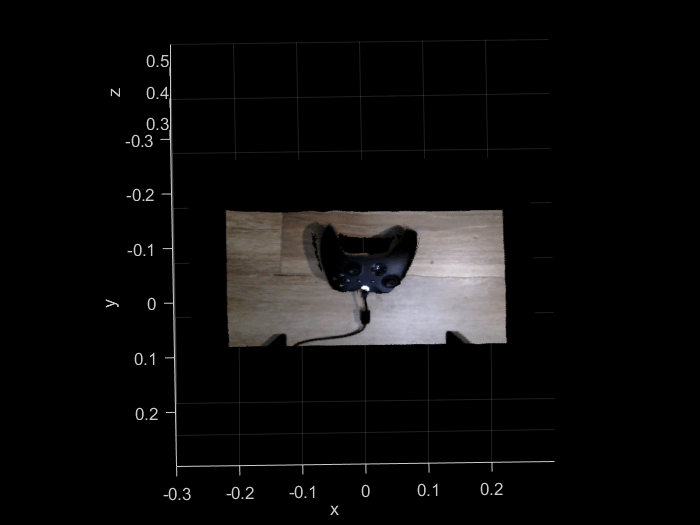# Generate Colorized Point Cloud Using Vision Module of Kinova Gen3 Robot

This example shows how to connect to the cameras of Kinova® Gen3 7-DoF Ultralightweight Robot and get the RGB and depth streams. Acquired images are utilized to create colorized point clouds of the environment.

Kinova robotics provides Image Acquisition Toolbox™ adaptor to access the camera streams. Ensure that you have completed the installation instructions for Image Acquisition Toolbox™ adaptor before proceeding with this example (for details, see Configure MATLAB to Acquire Images from Vision Module.

Clear all output in the live script by right clicking anywhere in the live script and then clicking ‘Clear All Output’ or navigate to VIEW > Clear all Output.

#### Products required

• Image Acquisition Toolbox

• Computer Vision Toolbox

• Robotics System Toolbox

### Connect to the Robot and Load Camera Calibration Parameters

To start working with the RGB and depth streams of the camera, you need to know the intrinsic and extrinsic parameters of the camera. The intrinsic parameters represent the optical center and focal length of the camera. The extrinsic parameters represent the location of the camera in the 3-D scene.

You can get initial values from the device manufacturer, but it is recommended to run a calibration routine to get the exact values of your device. In this example, you can use the values returned by the manipulator to generate the colorized point cloud from the RGB and Depth image.

` `

### Create an API instance to Connect to the Robot

```Simulink.importExternalCTypes(which('kortex_wrapper_data.h')); gen3Kinova = kortex(); gen3Kinova.ip_address = '192.168.1.10'; gen3Kinova.user = 'admin'; gen3Kinova.password = 'admin'; isOk = gen3Kinova.CreateRobotApisWrapper(); if isOk disp('You are connected to the robot!'); else error('Failed to establish a valid connection!'); end```
```You are connected to the robot! ```

### Get Intrinsic Parameters for RGB Camera

```[isOk, RGB_intrinsic] = gen3Kinova.GetIntrinsicParameters(1); if ~isOk error('Failed to acquire Intrinsic Parameters for RGB sensor.'); end```

### Get Intrinsic Parameters for Depth Camera

```[isOk, Depth_intrinsic] = gen3Kinova.GetIntrinsicParameters(2); if ~isOk error('Failed to acquire Intrinsic Parameters for depth sensor.'); end```

### Get Extrinsic Parameters

```[isOk, Extrinsic] = gen3Kinova.GetExtrinsicParameters(); if ~isOk error('Failed to acquire Extrinsic Parameters.'); end```

### Disconnect from the Robot

`isOk = gen3Kinova.DestroyRobotApisWrapper();`
```d_scalingFactor = 1000; % scaling factor [mm] d_R = [Extrinsic.rotation.row1.column1 , Extrinsic.rotation.row1.column2 , Extrinsic.rotation.row1.column3 ; Extrinsic.rotation.row2.column1 , Extrinsic.rotation.row2.column2 , Extrinsic.rotation.row2.column3; Extrinsic.rotation.row3.column1 , Extrinsic.rotation.row3.column2 , Extrinsic.rotation.row3.column3]; d_t = [Extrinsic.translation.x Extrinsic.translation.y Extrinsic.translation.z]; T = eye(4); T(1:3,1:3) = d_R; T(1:3,4) = d_t'; T = T'; d_extrinsics = T;```

### Connect to the Color Device

To access the RGB channel, create a video input object. A video input object represents the connection between MATLAB and a specific image acquisition device.` `

```vidRGB = videoinput('kinova_vision_imaq', 1, 'RGB24'); vidRGB.FramesPerTrigger = 1;```

Search all the video source objects associated with the video input object and return the video source object,

`srcRGB = getselectedsource(vidRGB);`

Modify the video source objects associated with the video input object

```srcRGB.Ipv4Address = gen3Kinova.ip_address; srcRGB.CloseConnectionOnStop = 'Enabled';```

### Connect to the Depth Device

Follow the same steps to connect to the depth stream of the RGB-D camera

```vidDepth = videoinput('kinova_vision_imaq', 2, 'MONO16'); vidDepth.FramesPerTrigger = 1; srcDepth = getselectedsource(vidDepth); srcDepth.Ipv4Address = gen3Kinova.ip_address; srcDepth.CloseConnectionOnStop = 'Enabled';```

### Get Snapshots from the RGB and Depth Streams

After connecting to the hardware, acquire images from the RGB and Depth camera. If you have already stored images on your computer, then load them in `depthData` and `rgbData` variables respectively.

```depthData = getsnapshot(vidDepth); rgbData = getsnapshot(vidRGB);```

Display Acquired Images

```figure; imshow(rgbData);`````` figure; h = imshow(depthData,hot); ax = h.Parent; h.CDataMapping = 'scaled'; ax.CLimMode = 'auto';```### Create a Colorized Point Cloud

Using the intrinsic parameters of both cameras and the extrinsic parameters, you can convert the RGB and depth streams to a colorized point cloud.

```[pcRaw, pcPnts,pcRGB] = pcfromgen3(depthData, rgbData, ... Depth_intrinsic.focal_length_x, Depth_intrinsic.focal_length_y, Depth_intrinsic.principal_point_x, Depth_intrinsic.principal_point_y, d_scalingFactor, d_extrinsics, ... RGB_intrinsic.focal_length_x, RGB_intrinsic.focal_length_y, RGB_intrinsic.principal_point_x, RGB_intrinsic.principal_point_y);```

Visualize Colorized Point Cloud

```pc = pointCloud(pcPnts, 'Color', pcRGB); figure; ax2 = pcshow(pc); axis(ax2, [-0.3, 0.3, -0.3, 0.3, 0.3, 0.6]); view(ax2, [1,-60]); xlabel('x');ylabel('y');zlabel('z');```### Close the Preview and Delete Video Input Objects

```if exist('vidRGB', 'var') if isvalid(vidRGB) closepreview(vidRGB); delete(vidRGB); end end if exist('vidDepth', 'var') if isvalid(vidDepth) closepreview(vidDepth); delete(vidDepth); end end```

Clear Workspace

`clear;`

### Function definition

```function [pcRaw, pcPnts, pcRGB] = pcfromgen3(depthData, rgbData,... d_fx, d_fy, d_ppx, d_ppy, d_scalingFactor, d_extrinsics,... rgb_fx, rgb_fy, rgb_ppx, rgb_ppy) % fx and fy: describe the focal length of the camera, as a multiple of pixel width and height % ppx and ppy: describe the pixel coordinates of the principal point (center of projection) % The center of projection is not necessarily the center of the image % Preallocate depthData= double(depthData); depthData(depthData == 0) = nan; [dh, dw] = size(depthData); % Convert to point cloud by applying depth intrinsics % (For each pixel the depth camera can be projected to the 3D space) pcRaw = zeros(dh,dw,3); pcRaw(:,:,3) = depthData/d_scalingFactor; [x,y] = meshgrid((1:dw),(1:dh)); pcRaw(:,:,1) = (x-d_ppx) .* pcRaw(:,:,3)/d_fx; pcRaw(:,:,2) = (y-d_ppy) .* pcRaw(:,:,3)/d_fy; % Transform the points to RGB frame by using rgb-depth extrinsics R = d_extrinsics(1:3, 1:3); t = d_extrinsics(4, 1:3); pcPnts = reshape(pcRaw,[],3) * R; %pcPnts = reshape(pcRaw,[],3) * R'; pcPnts(:,1) = pcPnts(:,1) + t(1); pcPnts(:,2) = pcPnts(:,2) + t(2); pcPnts(:,3) = pcPnts(:,3) + t(3); pcPnts = reshape(pcPnts, size(pcRaw)); % Create RGB associated with point cloud (registration) pcRGB = zeros(dh,dw,3,'uint8'); % Reproject each 3D point on the color image: Get indices into the RGB frame xind = (pcPnts(:,:,1) * rgb_fx)./ pcPnts(:,:,3) + rgb_ppx; yind = (pcPnts(:,:,2) * rgb_fy)./ pcPnts(:,:,3) + rgb_ppy; % Remove indices that are not visible to the RGB camera [rgbh, rgbw, ~] = size(rgbData); validInd = ~(xind < 1 | xind > rgbw | yind < 1 | yind > rgbh | isnan(xind) | isnan(yind)); % Simple approximation by rounding: xvalInd = round(xind(validInd)); yvalInd = round(yind(validInd)); rgbLinearInd = sub2ind([rgbh rgbw], yvalInd(:), xvalInd(:)); rgbDataTemp = uint8(rgbData(:,:,1)); color_temp = zeros(dh,dw,'uint8'); color_temp(validInd) = rgbDataTemp(rgbLinearInd); pcRGB(:,:,1) = color_temp; rgbDataTemp = uint8(rgbData(:,:,2)); color_temp = zeros(dh,dw,'uint8'); color_temp(validInd) = rgbDataTemp(rgbLinearInd); pcRGB(:,:,2) = color_temp; rgbDataTemp = uint8(rgbData(:,:,3)); color_temp = zeros(dh,dw,'uint8'); color_temp(validInd) = rgbDataTemp(rgbLinearInd); pcRGB(:,:,3) = color_temp; end ```